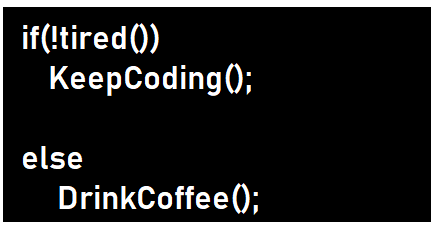# Reduce Array Size to The Half

## Introduction

This problem is one of the famous interview problems on HashTable and Sorting topics. Hashing is one of the most important algorithms. If you are coding in Java, then hashing is a must to learn. Sorting is essential to get the elements in ascending or descending order.

Reduce-array-size-to-half is the problem in which we have to find the maximum frequency and give the number of elements to be reduced or deleted.

We will see different approaches to solving this problem.

Let's understand the problem more clearly through the problem statement. So are you ready for this?

Source: Giphy

## Problem Statement

The problem we will cover in this blog is to Reduce the Array size to half, where we are given an array in which we have to remove the elements so that the array size reduces to half. If we pick an element, then all the occurrences of that element will be removed.

Let me explain this with an example.

Input: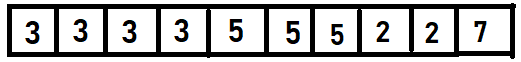Output:

2

As you can see, we have to pick the element which has occurred the maximum number of times. In this example, the array size is 10, and we have to make the array size 5 or less than 5. If we pick only 3, then the size of the array will become 6; for our condition to be fulfilled, we have to pick one more element. It can either be ‘2’, ’5’ or ‘7’. In total, we have to pick two elements, so that will be our output.

Our main work is to find the frequency to eliminate the elements to reduce the size of the array.

Note:

• The length of the array is even.

We will be heading towards our approach to building and coding for this problem.

### Approach 1

We will be using HashMap for storing the count. HashMap is a Map interface that stores the key and value pair where keys should be unique.

For more details regarding HashMap, do refer to these amazing, engaging articles→ Introduction to HashMap and Implementation of HashMap.

Input :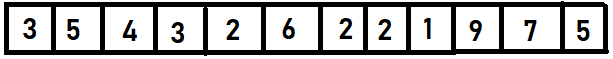Output:

3

As we see in the example, we have to keep the count of occurrences for every element; we will be using HashMap.  It will be stored like this: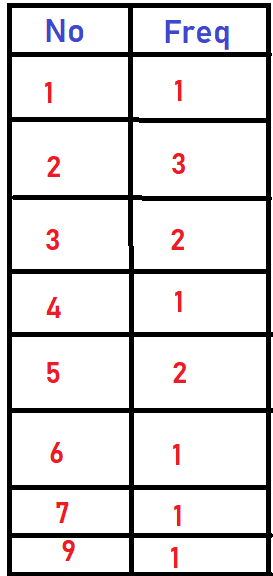To get the element having a maximum frequency, we will sort the array in descending order, due to which we will get to know which element is occurring most of the time.

After sorting table looks like this: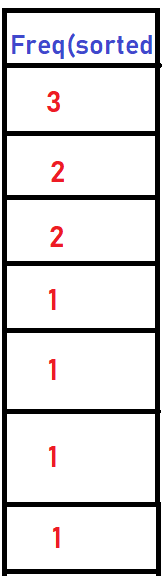• First, we will pick elements having a maximum frequency of ‘3’.
• Taking ans as a variable to store the number of elements to be deleted.
• Ans will be updated to 1 after selecting an element with frequency ‘3’.
• Now the size of the array will become ‘9,’ i.e., 12-3=9. We want the size to be ‘6’ or less than ‘6’.
• After frequency ‘3’, we will select the subsequent frequency from the table, i.e., ‘2’.
• Ans will be updated to 2 after selecting the element with frequency ‘2’.
• The size of the array will now become 9-2=7. It is still more than ‘6’.
• The subsequent frequency we will select is ‘2’.
• Ans will be updated to 3 after selecting the element with frequency ‘2’.
• The size of the array will become 7-2=5. Which is less than 6.
• The output will be 3.

#### Code

``````public class reduce {

public static int minSize(int[] arr,int n) {

//creating HashMap using its basic syntax
HashMap<Integer, Integer> count = new HashMap<>();
//storing the frequency along with the values using inbuilt functions of HashMap
for (int x : arr)
count.put(x, count.getOrDefault(x, 0) + 1);

//Creating array for storing the frequencies using counting sort
int[] counting = new int[count.values().size()];
int i=0;
for (int freq : count.values())
counting[i++]=freq;
Arrays.sort(counting);

int ans = 0, removed = 0, half = n / 2;
i=counting.length-1;
while (removed < half) {
ans ++;
removed+=counting[i--];
}
return ans;
}

//Main function
public static void main(String[] args) {
int arr[]= {3,3,3,3,5,5,5,2,2,7};
int n=10;
System.out.println(minSize(arr,n));

}

}``````

Output:

``````
2``````

#### Analysis of Complexity

Time ComplexityThe time complexity of finding the frequency and sorting the elements will be O(nlogn).

Space ComplexityThe space complexity is O(n) as we require extra space for storing the sorted elements.

### Approach 2

• In the worst case, the maximum value in the frequency array is n, which happens when all arr numbers are the same.
• We can sort frequencies by using Counting Sort, which is O(n) in Time Complexity.
• To solve this problem efficiently, we will be using some modifications to the previous approach.

#### Code

``````public class reduce {

public static int minSize(int[] arr,int n) {

//creating HashMap using its basic syntax
HashMap<Integer, Integer> count = new HashMap<>();
//storing the frequency along with the values using inbuilt functions of HashMap
for (int x : arr)
count.put(x, count.getOrDefault(x, 0) + 1);

//Creating array for storing the frequencies using counting sort
int[] counting = new int[n + 1];
for (int freq : count.values())
++counting[freq];

int ans = 0, removed = 0, half = n / 2, freq = n;
while (removed < half) {
ans += 1;
while (counting[freq] == 0) --freq;
removed += freq;
--counting[freq];
}
return ans;
}

//Main function
public static void main(String[] args) {
int arr[]= {3,3,3,3,5,5,5,2,2,7};
int n=10;
System.out.println(minSize(arr,n));

}

}``````

Output

``2``

#### Analysis of Complexity

Time Complexity: The time complexity will be O(n) as the counting sort is used.

Space Complexity: The space complexity is O(n).

### What do you mean by Counting Sort?

It is a sorting technique that counts the number of objects having distinct key values, i.e., hashing.

### What is the time complexity for finding the storing the frequency of elements?

O(n) time will be taken to find the frequency count using counting sort.

### What do you mean by HashMap?

HashMap allows us to store key and value pairs, where keys should be unique. It is like a Hashtable class but not synchronized. It may have one null key and multiple null values.

## Conclusion

This blog discusses finding the frequency of elements and eliminating their occurrences to reduce the array size to half. In our approaches, we have used Counting Sort and HashMap for solving the problem efficiently.

You can refer to this suggested article which will give you insights into questions about different Data Structures.

After solving this problem, you didn't feel excited to solve more problems based on Data Structures and Algorithms? But you might be unaware/dilemma about where to start from or what will be the roadmap to solve the problems on a variety of topics; Don't worry, Coding Ninjas has your back and will provide you with Problems, Sorting Based Problems, HashMap, and our paid courses, etc. You may also check out the mock test series and participate in the contests hosted on CodeStudio!

Also, if you are in the learning phase already, check out our new interview preparation and interview bundle for your placement preparations asked in big product-based companies like Amazon, Facebook, etc.